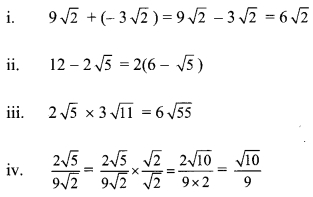# Maharashtra Board Class 9 Maths Solutions Chapter 2 Real Numbers Practice Set 2.3

## Maharashtra State Board Class 9 Maths Solutions Chapter 2 Real Numbers Practice Set 2.3

Question 1.
State the order of the surds given below.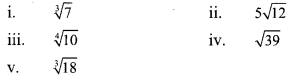i. 3, ii. 2, iii. 4, iv. 2, v. 3

Question 2.
State which of the following are surds Justify. [2 Marks each]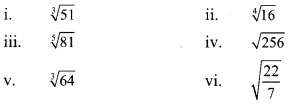i. $$\sqrt [ 3 ]{ 51 }$$ is a surd because 51 is a positive rational number, 3 is a positive integer greater than 1 and $$\sqrt [ 3 ]{ 51 }$$ is irrational.

ii. $$\sqrt [ 4 ]{ 16 }$$ is not a surd because= 2, which is not an irrational number.

iii. $$\sqrt [ 5 ]{ 81 }$$ is a surd because 81 is a positive rational number, 5 is a positive integer greater than 1 and $$\sqrt [ 5 ]{ 81 }$$ is irrational.

iv. $$\sqrt { 256 }$$ is not a surd because= 16, which is not an irrational number.

v. $$\sqrt [ 3 ]{ 64 }$$ is not a surd because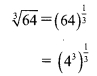= 4, which is not an irrational number.

vi. $$\sqrt { \frac { 22 }{ 7 } }$$ is a surd because $$\frac { 22 }{ 7 }$$ is a positive rational number, 2 is a positive integer greater than 1 and $$\sqrt { \frac { 22 }{ 7 } }$$ is irrational.

Question 3.
Classify the given pair of surds into like surds and unlike surds. [2 Marks each]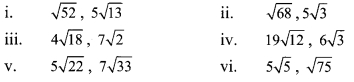Solution:
If the order of the surds and the radicands are same, then the surds are like surds.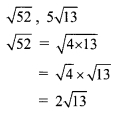Here, the order of 2$$\sqrt { 13 }$$ and 5$$\sqrt { 13 }$$ is same and their radicands are also same.
∴ $$\sqrt { 52 }$$ and 5$$\sqrt { 13 }$$ are like surds.Here, the order of 2$$\sqrt { 17 }$$ and 5$$\sqrt { 3 }$$ is same but their radicands are not.
∴ $$\sqrt { 68 }$$ and 5$$\sqrt { 3 }$$ are unlike surds.Here, the order of 12$$\sqrt { 2 }$$ and 7$$\sqrt { 2 }$$ is same and their radicands are also same.
∴ 4$$\sqrt { 18 }$$ and 7$$\sqrt { 2 }$$ are like surds.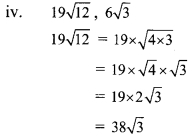Here, the order of 38$$\sqrt { 3 }$$ and 6$$\sqrt { 3 }$$ is same and their radicands are also same.
∴ 19$$\sqrt { 12 }$$ and 6$$\sqrt { 3 }$$ are like surds.

v. 5$$\sqrt { 22 }$$, 7$$\sqrt { 33 }$$
Here, the order of 5$$\sqrt { 22 }$$ and 7$$\sqrt { 33 }$$ is same but their radicands are not.
∴ 5$$\sqrt { 22 }$$ and 7$$\sqrt { 33 }$$ are unlike surds.Here, the order of 5√5 and 5√3 is same but their radicands are not.
∴ 5√5 and √75 are unlike surds.

Question 4.
Simplify the following surds.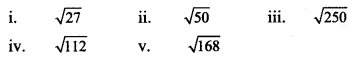Solution:Question 5.
Compare the following pair of surds.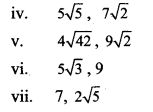Solution: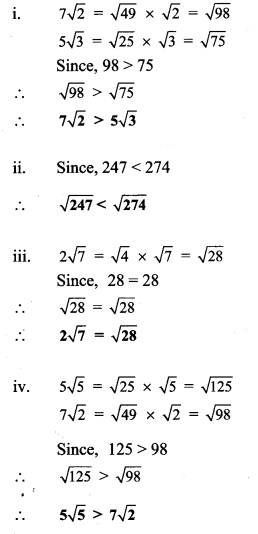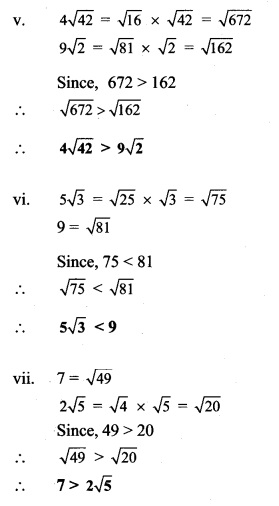Question 6.
Simplify.Solution: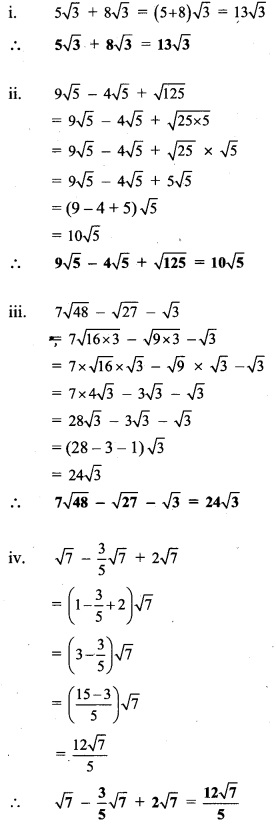Question 7.
Multiply and write the answer in the simplest form.Solution: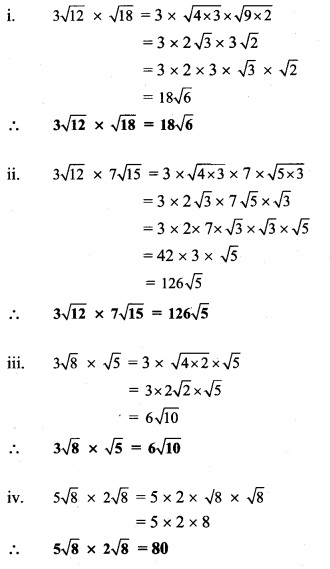Question 8.
Divide and write form.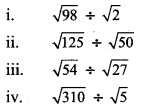Solution: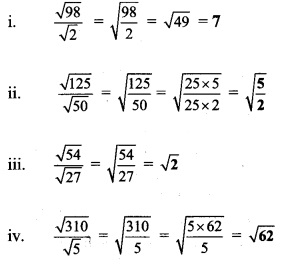Question 9.
Rationalize the denominator.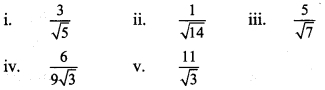Solution: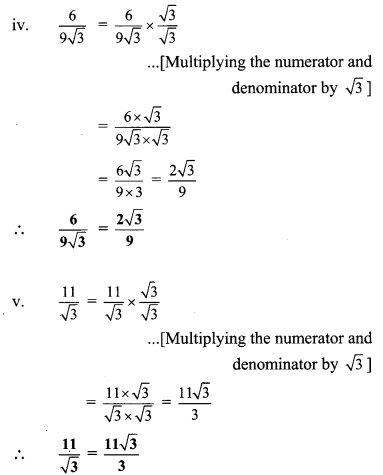Question 1.
$$\sqrt { 9+16 }$$ ? + $$\sqrt { 9 }$$ + $$\sqrt { 16 }$$ (Texbookpg. no. 28)
Solution:Question 2.
$$\sqrt { 100+36 }$$ ? $$\sqrt { 100 }$$ + $$\sqrt { 36 }$$ (Textbook pg. no. 28)
Solution:Question 3.
Follow the arrows and complete the chart by doing the operations given. (Textbook pg. no. 34)Solution:Question 4.
There are some real numbers written on a card sheet. Use these numbers and construct two examples each of addition, subtraction, multiplication and division. Solve these examples. (Textbook pg. no. 34)Solution: